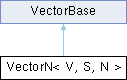MaterialX 1.38.8
Searching...
No Matches
VectorN< V, S, N > Class Template Reference

The class template for vectors of scalar values. More...

`#include <Types.h>`

Inheritance diagram for VectorN< V, S, N >:## Classes

class  Hash
Function object for hashing vectors. More...

## Public Types

using Iterator = typename std::array< S, N >::iterator

using ConstIterator = typename std::array< S, N >::const_iterator

## Public Member Functions

VectorN (Uninit)

VectorN (S s)

VectorN (const std::array< S, N > &arr)

VectorN (const vector< S > &vec)

VectorN (const S *begin, const S *end)

Comparison Operators
bool operator== (const V &rhs) const
Return true if the given vector is identical to this one.

bool operator!= (const V &rhs) const
Return true if the given vector differs from this one.

bool operator< (const V &rhs) const
Compare two vectors lexicographically.

Indexing Operators
S & operator[] (size_t i)
Return the scalar value at the given index.

const S & operator[] (size_t i) const
Return the const scalar value at the given index.

Component-wise Operators
operator+ (const V &rhs) const

VectorNoperator+= (const V &rhs)

operator- (const V &rhs) const
Component-wise subtraction of two vectors.

VectorNoperator-= (const V &rhs)
Component-wise subtraction of two vectors.

operator* (const V &rhs) const
Component-wise multiplication of two vectors.

VectorNoperator*= (const V &rhs)
Component-wise multiplication of two vectors.

operator/ (const V &rhs) const
Component-wise division of two vectors.

VectorNoperator/= (const V &rhs)
Component-wise division of two vectors.

operator* (S s) const
Component-wise multiplication of a vector by a scalar.

VectorNoperator*= (S s)
Component-wise multiplication of a vector by a scalar.

operator/ (S s) const
Component-wise division of a vector by a scalar.

VectorNoperator/= (S s)
Component-wise division of a vector by a scalar.

operator- () const
Unary negation of a vector.

Geometric Methods
getMagnitude () const
Return the magnitude of the vector.

getNormalized () const
Return a normalized vector.

dot (const V &rhs) const
Return the dot product of two vectors.

Iterators
Iterator begin ()

ConstIterator begin () const

Iterator end ()

ConstIterator end () const

Utility
S * data ()
Return a pointer to the underlying data array.

const S * data () const
Return a const pointer to the underlying data array.

## Static Public Member Functions

Static Methods
static constexpr size_t numElements ()
Return the number of scalar elements for the vector.

## Protected Attributes

std::array< S, N > _arr

## Detailed Description

template<class V, class S, size_t N>
class VectorN< V, S, N >

The class template for vectors of scalar values.

Inherited by Vector2, Vector3, Vector4, Color3, and Color4.

Template parameter V is the vector subclass, S is the scalar element type, and N is the number of scalar elements in the vector.

The documentation for this class was generated from the following file: